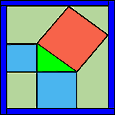# The Theorem, Loomis, and Different Proofs

The Pythagorean theorem states that for any right triangle
the area of the smallest square (AxA) plus (+)
the area of the middle sized square (BxB)
will always equal (=) the area of the largest square (CxC).
Thus (A2 + B2 = C2).

But this simple algebraic formula, while truthful, reveals nothing
of the elegant visual constructions devised for its demonstration.

Hundreds of proofs have been invented for this geometric proposition.
Dutifully collected by Elisha Scot Loomis in, The Pythagorean Proposition(1940),
this work records hundreds of proofs, and lends us a numbering system.
All the Loomis numbers (eg.,Loomis 09) cited here are from Part II, "The Geometric Proofs".
Since the Loomis figures are difficult to read, I have re-drawn and animated the proofs
using (for the most part) small multiples frames taken from a computer animation.

You have already "Beheld" one visual proof, Loomis 205, with Ganesha stepping thur it.
While this particular proof demonstrates itself with a single picture, most require
a series of pictures using color coding to replace the awkward identifing letters.
If you click on to the next few pages, you'll see ten more visual proofs.